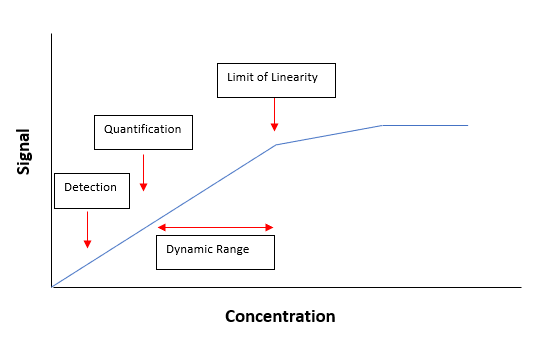# What are the definitions and differences of common Detection and Quantification Limits?

## Dokument-ID

Dokument-ID TE973

## Veröffentlichungsdatum

Veröffentlichungsdatum 06.04.2021
Frage
What are the definitions and differences of common Detection and Quantification Limits?
Zusammenfassung
Explanation of differences between Estimated Detection Limits (EDL), Method Detection Limits (MDL), and Practical Quantification Limits (PQL)
Antwort

Introduction:

Ranges for chemical measurements have limits. The lower limits are important because they identify whether a measurement is different from zero and if it can be quantified.

There are two distinct classes of reporting limits: detection limits and quantification limits. Detection limits refer to a minimum concentration of an analyte that can be detected. Quantification limits refer to a minimum concentration of an analyte that can be measured within specified limits of precision and accuracy. The following picture illustrates the relationship between detection and quantification (the example given below is for a linear calibration curve which has a limit of linearity):There are multiple kinds of detection and quantification limits. The most common ones used by Hach are defined below.

Please note that other detection and quantification limits exist. Definitions and procedures for determining these limits may vary. Customers should speak with their regulators to determine what limits are appropriate for them.

Estimated Detection Limit (EDL):

The lowest value stated in most Hach procedures is the EDL. These are generally based on the Sensitivity value for colorimetric methods. The EDL is the starting point when determining an MDL. It is also useful for comparing methods. The values are typically set via precision studies during the method development process, and/or during the instrument calibration for Hach methods. These tests are performed under ideal conditions, and current methods state that users may obtain different results.

The following article contains additional information on the Sensitivity Value:

Method Detection Limit (MDL):

The USEPA defines the MDL as the minimum concentration that can be found with a 99% level of confidence that the true concentration is higher than zero. A measurement below this MDL is highly suspect.

The idea is that any result below the MDL could be a false positive and could actually be zero. Also any result of zero could be a faulse negative and would better be stated as below the MDL as the actual concentration could be any value less than the MDL and still get a zero result.

The MDL is not fixed. It is different for each reagent lot, instrument, analyst, sample type, etc. Therefore, a published MDL may be a useful guide, but is only accurate for a specific set of circumstances. Each analyst should find a more accurate MDL for each specific sample matrix with the same equipment, reagents and standards that will routinely be used for measurements. Since the MDL is different from analyst to analyst, it is important that the MDL be found under actual operating conditions.

The MDL is a statistically calculated value based on the standard deviation of seven or more replicates at a concentration two to ten times the EDL, not at concentrations at or near the EDL. Also the recovery on the standards is not used in the calculation for the MDL. The concentration used can have an impact on the MDL. The MDL again is calculated based on the standard deviation, so the varience in results between replacates is what's used to determine MDL. If results are consistant, this can lead to low standard deviation and a low MDL. Generally higher concentrations will have more varience in concentration within the same percent tollerance and can lead to higher MDLs. So although it's counter intuitive, using a higher concentration standard to perform the MDL study can lead to higher more resonable MDLs.

The MDL value is calculated from the standard deviation of the replicate study results multiplied by the appropriate t value for a 99% confidence interval. For this definition, the MDL does not account for variation in sample composition and can only be achieved under ideal conditions.

1. Make an estimate of the detection limit. Use the EDL or sensitivity value stated in the Method Performance section fo the analysis procedure.
2. Prepare a laboratory standard of the analyte, two to ten times the EDL, in deionized (DI) water that is free of the analyte.
3. Make an analysis of at least seven portions of the laboratory standard and record each result.
4. Calculate the average and the standard deviation(s) of the results.
5. Calculate the MDL with the approate t value and the standard deviation value:  MDL = standard deviation x t-value

The following Table gives common t values:

 Number of replicates t Value 7 3.143 8 2.998 9 2.896 10 2.821

Practical Quantification Limit (PQL):

Sometimes referred to as the ML, the PQL is the lowest concentration that can be reliably achieved within specified limits of precision and accuracy during routine laboratory operating conditions. The PQL is usually calculated based on the MDL (so also specific to the instrument, operator, testing environment, ect.) and is specific to the tollerance and confidence interval.

Anhänge
Anhänge

## Verwandte Antworten

### Hat dir diese Antwort geholfen?

Wird gesendet...
Vielen Dank für Ihr Feedback.
Beim Senden ist ein Fehler aufgetreten. Versuchen Sie es erneut.